# JP4517793B2 - Permanent magnet synchronous motor control device and module - Google Patents

## Info

Publication number
JP4517793B2
JP4517793B2 JP2004266164A JP2004266164A JP4517793B2 JP 4517793 B2 JP4517793 B2 JP 4517793B2 JP 2004266164 A JP2004266164 A JP 2004266164A JP 2004266164 A JP2004266164 A JP 2004266164A JP 4517793 B2 JP4517793 B2 JP 4517793B2
Authority
JP
Japan
Prior art keywords
value
motor
axis
phase error
current
Prior art date
Legal status (The legal status is an assumption and is not a legal conclusion. Google has not performed a legal analysis and makes no representation as to the accuracy of the status listed.)
Active
Application number
JP2004266164A
Other languages
Japanese (ja)
Other versions
JP2006087152A (en
Inventor

Original Assignee

Priority date (The priority date is an assumption and is not a legal conclusion. Google has not performed a legal analysis and makes no representation as to the accuracy of the date listed.)
Filing date
Publication date
Application filed by 株式会社日立製作所 filed Critical 株式会社日立製作所
Priority to JP2004266164A priority Critical patent/JP4517793B2/en
Publication of JP2006087152A publication Critical patent/JP2006087152A/en
Application granted granted Critical
Publication of JP4517793B2 publication Critical patent/JP4517793B2/en
Active legal-status Critical Current
Anticipated expiration legal-status Critical

• 230000001360 synchronised Effects 0.000 title claims description 27
• 238000004364 calculation methods Methods 0.000 claims description 100
• 230000000875 corresponding Effects 0.000 claims description 12
• 230000000694 effects Effects 0.000 description 11
• 238000000034 methods Methods 0.000 description 1

## Images

•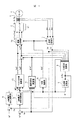•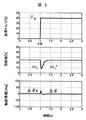•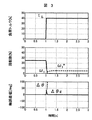•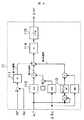•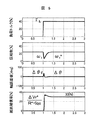•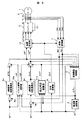•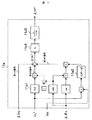•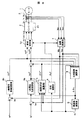•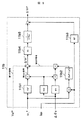•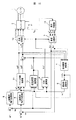•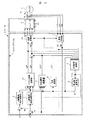## Classifications

• HELECTRICITY
• H02GENERATION; CONVERSION OR DISTRIBUTION OF ELECTRIC POWER
• H02PCONTROL OR REGULATION OF ELECTRIC MOTORS, ELECTRIC GENERATORS OR DYNAMO-ELECTRIC CONVERTERS; CONTROLLING TRANSFORMERS, REACTORS OR CHOKE COILS
• H02P21/00Arrangements or methods for the control of electric machines by vector control, e.g. by control of field orientation
• H02P21/04Arrangements or methods for the control of electric machines by vector control, e.g. by control of field orientation specially adapted for very low speeds
• HELECTRICITY
• H02GENERATION; CONVERSION OR DISTRIBUTION OF ELECTRIC POWER
• H02PCONTROL OR REGULATION OF ELECTRIC MOTORS, ELECTRIC GENERATORS OR DYNAMO-ELECTRIC CONVERTERS; CONTROLLING TRANSFORMERS, REACTORS OR CHOKE COILS
• H02P21/00Arrangements or methods for the control of electric machines by vector control, e.g. by control of field orientation
• H02P21/24Vector control not involving the use of rotor position or rotor speed sensors
• H02P21/26Rotor flux based control

## Description

The present invention relates to a position sensorless vector control system for a permanent magnet synchronous motor.

As a resistance identification technique of the position sensorless vector control method, as described in Japanese Patent Application Laid-Open No. 2003-164188, before starting the operation of the motor, an inverter voltage is applied so as to restrain the motor, and the voltage flows. In the method of identifying the resistance value from the restraint current and during operation, a means for identifying the counter electromotive voltage coefficient of the motor is provided, and the value identified during operation and the preset counter electromotive voltage coefficient at normal temperature, A method is described in which the motor temperature is estimated and the resistance value is identified using the estimated temperature value.

JP 2003-164188 A

When high torque is required during speed control or torque control operation, it is necessary to flow a large current commensurate with the torque. When high torque is required in continuous time, the resistance value R of the motor increases with time due to heat generated by the motor current.

In the position sensorless control, for example, an error value (hereinafter referred to as a phase error value Δθ) between the “control shaft reference rotation phase command value θc * ” and the “motor shaft reference rotation phase value θ” is estimated by calculation, The frequency command value ω 1 * is adjusted so that the estimated phase error value Δθc becomes “zero”.
The rotation phase command value θc * is created by integrating ω 1 * .

Thus, in order to calculate the inverter voltage command values Vd ** and Vq ** and the estimated phase error value Δθc, it is necessary to set the motor resistance value R.

If a setting error (R-R * ) occurs between the set value R * and the motor resistance value R, if an impact load disturbance or the like enters in the low speed rotation range, the actual phase error value Δθ and the estimated phase error There is a case in which the value Δθc is deviated, and as a result, the optimum phase cannot be controlled, resulting in an inoperable state.

However, in the method described in Japanese Patent Application Laid-Open No. 2003-164188, it is described that the identification calculation of the back electromotive force coefficient needs to be performed in a region where the load torque is small, and there is a problem that the accuracy of the calculation decreases in a high torque region. Yes.

For this reason, the estimation accuracy of the motor temperature also deteriorates during high torque operation, and as a result, it is impossible to realize “highly accurate resistance identification”.

An object of the present invention is to provide a “permanent magnet motive motor vector control device” capable of realizing “high-precision position sensorless vector control” even in a high torque range.

The present invention identifies an equivalent of a motor resistance value or a resistance setting error using an output value equivalent to current control, a current detection value, a frequency command value, an estimated phase error value, and a motor constant, and uses this identification value. Thus, the set value R * equivalent of the voltage command calculation unit and the phase error calculation unit is corrected (corrected).

It is possible to provide a “vector control device for a permanent magnet motor” that can realize “high-precision position sensorless vector control” even in a high torque range.

Hereinafter, embodiments of the present invention will be described in detail with reference to the drawings.

(First embodiment)
FIG. 1 shows an example of the configuration of a vector control apparatus for a permanent magnet motor according to an embodiment of the present invention.

1 is a permanent magnet synchronous motor, 2 is an inverter that outputs a voltage proportional to the voltage command values Vu * , Vv * , and Vw * of a three-phase AC, 21 is a DC power source, and 3 is a three-phase AC current Iu, Iv, Iw. A detectable current detector 4 is a phase error calculation unit that calculates an estimated value (Δθc) of a phase error value Δθ (= θc * −θ) that is a deviation between the rotation phase command value θc * and the rotation phase value θ of the motor 1. Reference numeral 5 denotes a frequency calculation unit that calculates the frequency command value ω 1 * from the estimated phase error value Δθc, 6 denotes a phase calculation unit that calculates the rotational phase command θc * of the motor 1 from the frequency command value ω 1 * , and 7 denotes the above-mentioned 3 Phase alternating current Iu,
A coordinate conversion unit that outputs the detected current values Idc and Iqc of the d-axis and the q-axis from the detected values Iuc, Ivc and Iwc of the Iv and Iw and the rotation phase command value θc * , and 8 is a first d-axis current given from the host A d-axis current command calculation unit that outputs a second d-axis current command value Id ** in accordance with the deviation between the command value Id * and the detected d-axis current value Idc, 9 is a first q-axis current given from the host A q-axis current command calculation unit 10 outputs a second d-axis current command value Iq ** in accordance with a deviation between the command value Iq * and the q-axis current detection value Iqc. Using the command values Id ** and Iq ** , the frequency command value ω 1 *, and the electric constants of the motor 1, a vector control calculation unit 11 that outputs the voltage command values Vd ** and Vq ** , 11 is the second q axis current command value Iq ** and the q-axis current detection value Iqc and the frequency command value omega 1 * and Oyo estimated phase error value Δθc Based on the electrical constant of the motor 1, the voltage value? Vr ^ including the setting errors of resistance were identified, using the identified values, performs a proportional or integral calculation, to calculate the setting of the resistance error voltage? Vr *, q A resistance identification calculation unit 12 outputs to the calculation unit of the shaft voltage command value Vq *** and the estimated phase error value Δθc, 12 is a three-phase alternating current from the voltage command values Vd ** and Vq *** and the rotation phase command value θc * This is a coordinate conversion unit for outputting voltage command values Vu * , Vv * , Vw * .

First, the basic operation of the voltage control and phase control of the position sensorless vector control method when the “resistance identification calculation unit 11”, which is a feature of the present invention, is not provided (ΔVr * is “zero”) will be described.

The basic operation of the voltage control is based on vector control using the first current command values Id * and Iq * and the current detection values Idc and Iqc given from the host in the d-axis and q-axis current command calculation units 8 and 9. Second intermediate current command values Id ** and Iq ** used for calculation are calculated.

The vector control calculation unit 10 uses the second current command values Id ** , Iq ** , the frequency command value ω 1 *, and the motor constant setting value, and the voltage command values Vd ** , Vq ** is calculated, and inverter voltage command values Vu * , Vv * , Vv * are controlled.

Where R: resistance value, Ld: d-axis inductance value, Lq: q-axis inductance value,
Ke: induced voltage coefficient, *: set value.

On the other hand, regarding the basic operation of phase control, the phase error calculation unit 4 uses the voltage command values Vd ** , Vq ** , the current detection values Idc, Iqc, the frequency command value ω 1 *, and the set value of the motor constant. The phase error value Δθ (= θc * −θ), which is the deviation between the rotational phase command value θc * and the rotational phase value θ, is estimated. The calculation of the estimated phase error value Δθc is the number (2).

Further, the frequency calculation unit 5 controls the frequency command value ω 1 * by the calculation shown in Equation (3) so that the estimated phase error value Δθc becomes “zero”.

Here, Kp: proportional gain, Ki: integral gain.

The phase calculating unit 6, using a frequency command value omega 1 *, to control the rotational phase command value .theta.c * in operation shown in Formula (4).

The above is the basic operation of the conventional voltage control and phase control.

Next, the effect of using the “resistance identification calculation unit 11”, which is a feature of the present invention, will be described.

FIG. 2 and FIG. 3 show simulation characteristics when an impact load is applied in the case where the resistance setting error voltage value ΔVr * is not added in the control device of FIG.

FIG. 2 shows characteristics when the resistance value R of the motor matches the set value R * set in the phase difference calculation unit 4 and the vector control calculation unit 10 (R = R * ).

In a control configuration in which a speed control system is added to the host system, when the motor is operating at a constant speed of 25 [%] in the low speed range, impact load τ L is entered at point A after time 1 [s]. Then, the number of rotations of the motor rapidly decreases to near 10 [%] speed.

At this time, the actual phase error value Δθ (θc * −θ) and the estimated phase error value Δθc coincide with each other, and the rotational frequency ω 1 and the frequency command value ω 1 * are also stably recovered and the state can be seen.

However, when the resistance value R and the set value R * of the motor in FIG. 3 do not match (R = 1.3 × R * ), the rotational frequency ω 1 decreases to “zero” at the time of impact load, and the frequency It can be seen that the command value ω 1 * is a value larger than “zero” and is constantly settled (referred to herein as an inoperable state). At this time, it can be seen that the actual phase error value Δθ (θc * −θ) and the estimated phase error Δθc do not match.

In other words, if there is a resistance setting error (R−R * ) in the phase error calculation unit 4 and the vector control calculation unit 10 in the low rotation speed range, an operation impossible state may occur during an impact load. .

Here, the “principle for identifying resistance value” which is a feature of the present invention will be described.

The vector control calculation unit 10 calculates voltage command values Vd ** and Vq ** represented by the number (5) obtained by rewriting the number (1).

When a phase error value Δθ which is a deviation between θc * which is the reference of the control axis and θ of the motor reference is generated, the coordinate transformation matrix from the control axis (dc−qc) to the motor axis (dq) is a number ( 6).

When the above-described phase error value Δθ occurs, the applied voltages Vd and Vq of the motor calculated on the control side become the number (7) from the numbers (5) and (6).

On the other hand, the applied voltages Vd and Vq of the motor are estimated phase error value Δθc, current detection value Idc,
Expressed using Iqc, motor constant,

Here, the relationship of the right side of number (7) = number (8), and Lq * = Lq, Ld * = Ld, Ke * =
When the current command value is calculated by setting Ke and Id * to “zero” and Iq * to “predetermined value”, the output value Iq ** of the q-axis current command calculation unit 9 is expressed by the number (9). Can show.

In addition, in the number (9), the relationship of the number (10) is established at the low rotation speed.

Then, the number (9) can be approximated as the number (11).

Here, by multiplying both sides of the equation (11) by the resistance setting value R * and subtracting the voltage value of R * × Iqc,

When the voltage value ΔVr (= (R−R * ) × Iqc) including the resistance setting error is obtained from the equation (12),

Therefore, by calculating the number (14) using the second q-axis current command value Iq ** , the current detection value Iqc, the frequency command value ω 1 * , the estimated phase error value Δθc, and the motor constant, The value ΔVr can be identified.

Next, the “resistance value setting error correction method” using the identified voltage value ΔVr ^ will be described. In the operation of voltage control, if a signal obtained by multiplying the voltage value ΔVr ^ calculated by the equation (14) by a proportional gain Kv is ΔVr * ,

The number (15) is added to the q-axis voltage command value Vq ** to make the new voltage command value Vq *** .

Here, when the phase error value Δθ occurs in the state where the voltage value ΔVr * is added,

The applied voltages Vd and Vq of the motor are expressed using motor constants (rewriting Equation 8).

From the relationship of the right side of the number (17) = number (18), the output value Iq ** of the q-axis current command calculation unit 9 can be approximated by the number (19) in the low speed range.

Substituting the second q-axis current command value Iq ** obtained in Equation (19) into Equations (14) and (15) yields Equation (20).

Furthermore, in the equation (20), when the proportional gain Kv is set larger than 1

From equation (21), the voltage value ΔVr equivalent including the resistance setting error can be supplied from the output value ΔVr * of the resistance identification calculation unit 11.

Here, the “resistance identification calculation unit 11”, which is a feature of the present invention, will be described with reference to FIG.

Basically, the resistance identification calculation unit 11 calculates the number (14), and the motor resistance set value 111 is added to the deviation between the second q-axis current command value Iq ** and the q-axis current detection value Iqc. Is multiplied by three, the value obtained by subtracting the cosine signal of the estimated phase error value Δθc from the constant “1”, the frequency command value ω 1 *, and the setting value 112 of the induced voltage coefficient. And a third signal obtained by multiplying the sine (sin) signal of the estimated phase error value, the square value of the frequency command value, and the constant calculation value 113 of the motor. to add.

The result obtained by multiplying the added value by the proportional gain 114 is input to the primary delay filter 115 corresponding to the current control delay time, and the output value becomes a voltage value ΔVr * that compensates for the resistance setting error.

Also in the phase control operation, “resistance value setting error correction” is performed using the voltage value ΔVr * . In the phase error calculation unit 4 of FIG. 1, using the voltage command values Vd ** , Vq *** , the current detection values Idc, Iqc, the frequency command value ω 1 * , the set value of the motor constant and the voltage value ΔVr * , An estimation calculation of the phase error value Δθ is performed.

The calculation of the estimated phase error value Δθc is the number (22).

From the equations (21) and (22), the estimated phase error value Δθc is

As a result, even if the set value R * set in the phase error calculation unit 4 and the vector control calculation 10 does not coincide with the resistance value R of the motor, Iq which is the output value of the q-axis current command calculation unit 9 ** can be used to correct the resistance setting error.

FIG. 5 shows a simulation waveform to which this embodiment is applied (R = 1.3 × R * ).

Even when the resistance value R and the set value R * do not match, it can be seen that by compensating the voltage value ΔVr * , the motor does not fall into an inoperable state and is stably controlled.

The “resistance compensation rate” shown fourth from the top indicates a ratio of “voltage value ΔVr * for correcting resistance setting error” and “multiplied value of R * and Iqc”.

It can be seen that the resistance setting error equivalent to 30 [%] is corrected faithfully.

In this embodiment, a signal obtained by multiplying the identified voltage value ΔVr ^ by the proportional gain Kv is set as ΔVr * . However, it is obvious that the same effect can be obtained even when a signal obtained by integrating the voltage value ΔVr ^ is set as ΔVr *. It is.

In this embodiment, the voltage value ΔVr * is used as a voltage value for correcting the resistance setting error. However, ΔVr * is divided by Iqc or Iq * to set the resistance setting error value (R−R * ). It is clear that the same effect can be obtained by directly obtaining and adding directly to the set values of the phase error calculation unit 4 and the vector control calculation unit 10.

(Second embodiment)
FIG. 6 shows another embodiment of the present invention.

The present embodiment is a vector control device for a permanent magnet same-machine motor that corrects the output values Vd * and Vq * of the vector control calculation with the deviation between the current command value and the current detection value given from the host.

In FIG. 6, 1 to 7, 12, and 21 are the same as those in FIG.

8a is a d-axis current control calculation unit that calculates ΔVd so that the d-axis current command value Id * and the d-axis current detection value Idc match, and 9a the q-axis current command value Iq * and the q-axis current detection value Iqc match. The q-axis current control calculation unit 10a for calculating ΔVq in such a manner uses the d-axis and q-axis current command values Id * and Iq * , the frequency command value ω 1 *, and the motor constant setting value to A vector control calculation unit 11a for outputting reference values Vd * and Vq * , 11a is a q-axis current control output value ΔVq, a q-axis current detection value Iqc, a frequency command value ω 1 * , an estimated phase error value Δθc, and a motor constant setting value Is a resistance identification calculation unit that identifies a voltage value ΔVr * equivalent including a resistance setting error.

The difference from FIG. 1 shown in the previous embodiment is that, in the d-axis and q-axis current control calculation units 8a and 9a, the current detection values Idc and Iqc are added to the current command values Id * and Iq * given from the upper level. The voltage correction values ΔVd and ΔVq are calculated so as to coincide.

Further, the vector control calculation unit 10a uses the current command values Id * and Iq * , the frequency command value ω 1 * and the motor constant setting value to obtain the voltage command reference values Vd * and Vq * shown in the equation (24). Operate,

As shown in the equation (25), the inverter voltage command values Vd ** and Vq ** are calculated.

Next, the effect which this invention brings about is demonstrated.

First, when ΔVr * = 0 and a phase error value Δθ is generated, the voltage command values Vd ** and Vq ** are the number (26).

Also, the applied voltages Vd and Vq of the motor are expressed using the phase error value Δθ and the motor constant (Equation 8 is rewritten),

Here, the relationship of the right side of number (26) = number (27), Lq * = Lq, Ld * = Ld, Ke * = Ke, and Id * are set to “zero”, and Iq * is set to “predetermined value”. When the current control is performed, the output value ΔVq of the q-axis current control calculation unit 9a can be expressed by the number (28).

Here, when the term of the voltage value ΔVr (= (R−R * ) × Iqc) including information on the resistance setting error is arranged,

By calculating the number (30) using the output value ΔVq of the q-axis current control, the frequency command value ω 1 * , the estimated phase error value Δθc and the set value of the motor constant from the number (29), the voltage value ΔVr can be identified.

Next, the “resistance value setting error correction method” using the voltage value ΔVr ^ will be described.

When a signal obtained by multiplying the voltage value ΔVr ^ calculated by the number (30) by the proportional gain Kv is ΔVr * ,

Here, the number (31) is added to the q-axis voltage command value Vq ** ,

When used in the calculation of the estimated phase error value Δθc, as in the first embodiment, the voltage value ΔVr equivalent including the resistance setting error can be supplied from the output value ΔVr * of the resistance identification calculation unit 11a.

The present invention can also be applied to a vector control apparatus for a permanent magnet motor that corrects an output of a vector control calculation based on a deviation between a current command value and a current detection value.

Here, the resistance identification calculation unit 11a, which is a feature of the present invention, will be described with reference to FIG.

Basically, the resistance identification calculation unit 11a performs the calculation of the number (30). From the first signal that is the output value ΔVq of the q-axis current control and the constant “1”, the estimated phase error value Δθc is calculated. The second signal obtained by multiplying the cosine (cos) signal cosΔθc by three values, the frequency command value ω 1 * and the induced voltage coefficient setting value 11a1, and the sine signal of the estimated phase error value
It is obtained by multiplying sinΔθc, cosine (cos) signal cosΔθc, frequency command value ω 1 * , q-axis current detection value Iqc, and 11a2, which is the difference value between the d-axis inductance value and q-axis inductance value of the motor. The third signal is added.

The result obtained by multiplying this added value by the proportional gain 11a4 is input to the primary delay filter 11a5 corresponding to the current control delay time, and this output value becomes the voltage value ΔVr * for compensating for the resistance setting error.

In this embodiment, a signal obtained by multiplying the identified voltage value ΔVr ^ by the proportional gain Kv is set as ΔVr * . However, it is obvious that the same effect can be obtained even when a signal obtained by integrating the voltage value ΔVr ^ is set as ΔVr *. It is.

In this embodiment, the voltage value ΔVr * is used as a voltage value for correcting the resistance setting error. However, ΔVr * is divided by Iqc or Iq * to set the resistance setting error value (R−R * ). It is obvious that the same effect can be obtained by directly obtaining and adding directly to the set values of the phase error calculation unit 4 and the vector control calculation unit 10a.

(Third embodiment)
FIG. 8 shows another embodiment of the present invention.

The present embodiment is a vector controller for a permanent magnet motor that directly controls the d-axis and q-axis voltage command values Vd ** and Vq ** by the deviation between the current command value and the current detection value given from the host. is there.

In FIG. 8, 1 to 7, 12, and 21 are the same as those in FIG. 8b is a d-axis current control calculation unit that controls the d-axis voltage command value Vd ** so that the d-axis current command value Id * and the d-axis current detection value Idc match, and 9b is the q-axis current command value Iq * and q. The q-axis current control calculation unit 11b controls the q-axis voltage command value Vq ** so that the detected shaft current values Iqc coincide with each other, 11b includes the q-axis voltage command value Vq ** , the q-axis current detection value Iqc, and the frequency command value ω. A resistance identification calculation unit that outputs ΔVr * corresponding to a voltage value ΔVr including a resistance setting error using 1 * , an estimated phase error value Δθc, and a motor constant setting value.

The difference between FIG. 1 shown in the previous embodiment, the d-axis and q-axis current control calculation section 8b, 9b, the first current command value Id provided from the upper *, Iq * to the current detection value Idc, The voltage command values Vd ** and Vq ** are calculated so that Iqc matches.

Next, the effect which this invention brings about is demonstrated.

First, the applied voltages Vd and Vq of the motor are expressed by using the phase error value Δθ and the motor constant (rewriting Equation 8),

Here, the relationship between the voltage command values Vd ** and Vq ** and the right side of the number (33), Lq * = Lq, Ld * = Ld, Ke * = Ke, and Id * are set to “zero”, Iq *. Is set to a “predetermined value” and current control is performed, the output value Vq ** of the q-axis current control calculation unit 9b can be expressed by the number (34).

Here, when the term of the voltage value ΔVr (= R × Iqc) including information on the motor resistance value is arranged,

From the equation (35), by calculating the equation (36) using the output value Vq ** of the q-axis current control, the frequency command value ω 1 * , the estimated phase error value Δθc, and the motor constant setting value, The voltage value ΔVr can be identified.

Next, the “resistance value correction method” using the voltage value ΔVr ^ will be described.

A voltage value that is a product of the signal obtained by multiplying the voltage value ΔVr ^ calculated by the equation (36) by the proportional gain Kv, the resistance setting value R * set in the phase error calculation unit 4 and the q-axis current detection value Iqc. If the signal obtained by adding and is ΔVr * ,

The new q-axis voltage command value Vq ***

Further, when Vq *** is used for the calculation of the estimated phase error value Δθc, the voltage value ΔVr equivalent including the resistance value can be supplied from the output value ΔVr * of the resistance identification calculation unit 11b.

The present invention can also be applied to a permanent magnet same motor vector control device that outputs a vector control calculation based on a deviation between a current command value and a current detection value.

Here, the “resistance identification calculation unit 11b”, which is a feature of the present invention, will be described with reference to FIG. In the resistance identification calculation unit 11b, the first signal that is the output value Vq ** of the q-axis current control, the cosine (cos) signal of the estimated phase error value Δθc, the frequency command value ω 1 *, and the setting value of the induced voltage coefficient The second signal obtained by multiplying three of 11b1 and the sine signal of the estimated phase error value Δθc.
sinΔθc, cosine (cos) signal cosΔθc, frequency command value ω 1 * , q-axis current detection value Iqc, and third value obtained by multiplying the difference value 11b2 between the d-axis inductance value and q-axis inductance value of the motor. Are added. The result obtained by multiplying this added value by the proportional gain 11b4 is input to the primary delay filter 11b5 corresponding to the current control delay time. Further, from the output value, the product of the q-axis current detection value Iqc and the resistance set value 11b3 is obtained. The voltage value ΔVr * is subtracted to compensate for the resistance setting error.

In this embodiment, a signal obtained by multiplying the identified voltage value ΔVr ^ by the proportional gain Kv is set as ΔVr * . However, it is obvious that the same effect can be obtained even when a signal obtained by integrating the voltage value ΔVr ^ is set as ΔVr *. It is.

In this embodiment, the voltage value ΔVr * is used as a voltage value including a resistance setting error (R−R * ). However, the voltage value ΔVr * is divided by Iqc or Iq * to obtain a setting error value ( The same effect can be obtained by directly obtaining (R−R * ) and adding it directly to the set value R * of the phase error calculation unit 4.

(Fourth embodiment)
In the above first to third embodiments,
Although the system detects the three-phase alternating currents Iu to Iw detected by the expensive current detector 3, the present invention can also be applied to a control device that performs inexpensive current detection.

FIG. 10 shows this embodiment.

10, components 1, 2, 4 to 12, 21 are the same as those in FIG.

13 is a current reproduction unit which reproduces from the DC current I DC flowing through the input bus of the inverter 2, alternating currents Iu 3 phase flowing to the motor 1, Iv, and Iw.

Using the estimated current values Iu ^, Iv ^, Iw ^, the coordinate conversion unit 7 calculates the detected current values Idc, Iqc for the d-axis and the q-axis.

Even in such a current sensorless control system, since Id * and Idc, and Iq * and Iqc coincide with each other, it is apparent that the operation is the same as that of the embodiment of FIG. 1 and the same effect is obtained.

In this embodiment, the embodiment shown in FIG. 1 is used. However, the same effect can be obtained by using the embodiment shown in FIGS.

(Fifth embodiment)
An example in which the present invention is applied to a module will be described with reference to FIG. This example shows an embodiment of the first example. Here, the phase error calculation unit 4, the frequency calculation unit 5, the phase calculation unit 6, the coordinate conversion unit 7, the d-axis current command calculation unit 8, the q-axis current command calculation unit 9, the vector control calculation unit 10, the resistance identification calculation unit 11. The coordinate conversion unit 12 is configured using a one-chip microcomputer. The one-chip microcomputer and the power converter (inverter 2) are housed in one module configured on the same substrate. The module here means “standardized structural unit” and is composed of separable hardware / software components. In addition, although it is preferable that it is comprised on the same board | substrate on manufacture, it is not limited to the same board | substrate. From this, it may be configured on a plurality of circuit boards built in the same housing. In other embodiments, the same configuration can be adopted.

As described above, according to the present invention, it is possible to realize control characteristics that are robust against changes in the resistance constant of the motor in the low rotational speed region of position sensorless control. In addition, a “permanent magnet synchronous motor vector control device” that can be commonly used can be provided even in a system that performs inexpensive current detection.

Further, even during actual operation, highly accurate vector control can be realized by identifying the equivalent resistance value of the motor and automatically correcting the resistance constant set in the control system.

An example of a block diagram of the vector control apparatus of the permanent magnet synchronous motor which shows one Example of this invention. An example of an impact load characteristic diagram (R = R * ) when there is no resistance identification calculation unit 11. An example of an impact load characteristic diagram (R = 1.3R * ) when the resistance identification calculation unit 11 is not provided. An example of the resistance identification calculating part 11 in the control apparatus of FIG. An example of an impact load characteristic diagram (R = 1.3R * ) when the resistance identification calculation unit 11 is inserted. The block diagram of the vector control apparatus of the permanent magnet synchronous motor which shows the other Example of this invention. An example of the resistance identification calculating part 11a in the control apparatus of FIG. The block diagram of the vector control apparatus of the permanent magnet synchronous motor which shows the other Example of this invention. An example of the resistance identification calculating part 11b in the control apparatus of FIG. An example of the vector control apparatus of the permanent magnet synchronous motor which shows the other Example of this invention. The figure of the Example which applied this invention to the module.

Explanation of symbols

DESCRIPTION OF SYMBOLS 1 ... Permanent magnet synchronous motor, 2 ... Inverter, 3 ... Current detector, 4 ... Phase error calculating part, 5 ... Frequency calculating part, 6 ... Phase calculating part, 7 ... Coordinate converting part, 8 ... d-axis current command calculating part , 9 ... q-axis current command calculation unit, 8a, 8b ... d-axis current control calculation unit, 9a, 9b ... q-axis current control calculation unit, 10, 10a, 10b ... vector control calculation unit, 11, 11a, 11b ... resistance Identification calculation unit,
DESCRIPTION OF SYMBOLS 12 ... Coordinate conversion part, 13 ... Current reproduction part, Id * ... 1st d-axis current command value, Id ** ... 2nd d-axis current command value, Iq * ... 1st q-axis current command value, Iq ** … Second q-axis current command value,
ΔVd: d-axis current control output value, ΔVq: q-axis current control value output value, Vd ** : d-axis voltage command value, θc * : rotational phase command value, ω 1 * ... frequency command value, Δθ ... Phase error value, Δθc: Estimated phase error value.

## Claims (9)

1. A phase error value that is a deviation between the rotational phase command value obtained by integrating the frequency command value of the inverter that controls the permanent magnet synchronous motor and the rotational phase value of the permanent magnet motor is obtained, and the estimated phase error value becomes zero. In the vector controller of the permanent magnet synchronous motor that calculates the frequency command value as follows:
The d-axis (corresponding to the magnetic flux axis) and q-axis (corresponding to the torque axis) current value command value or current detection value of the rotating coordinate system, and the current detection value of the motor follow the current command values of the d-axis and q-axis that are given from the top. Using the current control output value, frequency command value, estimated phase error value, and motor constant calculated to control the motor resistance value ,
The motor resistance value identification calculation is performed by multiplying the first signal that is the output value of the q-axis current control, the cosine signal of the estimated phase error value, the frequency command value, and the induced voltage coefficient of the motor. The obtained second signal, an estimated phase error value sine signal, an estimated phase error value cosine signal, a frequency command value, a q-axis current detection value, a motor d-axis inductance value and q A control device for a permanent magnet synchronous motor, wherein the control is performed by adding a third signal obtained by multiplying a difference value of shaft inductance values .
2. In the control device of the permanent magnet synchronous motor according to claim 1,
By dividing the resistance value of the motor obtained by the identification calculation by proportional or integral calculation and using the output value subjected to the first-order lag processing, by the q-axis current command value or the q-axis current detection value, A control device for a permanent magnet synchronous motor, wherein a resistance value of a motor or a setting error value of the resistance is obtained by calculation, and a setting value of the resistance in at least one of a q-axis voltage command value or a phase error value is corrected .
3. A phase error value that is a deviation between the rotational phase command value obtained by integrating the frequency command value of the inverter that controls the permanent magnet synchronous motor and the rotational phase value of the permanent magnet motor is obtained, and the estimated phase error value becomes zero. In the vector controller of the permanent magnet synchronous motor that calculates the frequency command value as follows:
The d-axis (corresponding to the magnetic flux axis) and q-axis (corresponding to the torque axis) current value command value or current detection value of the rotating coordinate system and the current detection value of the motor follow the d-axis and q-axis current command values given from the top. Using the current control output value, frequency command value, estimated phase error value, and motor constant calculated to control the motor resistance value,
The motor resistance value identification calculation is performed by subtracting the first signal which is the output value of the q-axis current control and the cosine signal of the estimated phase error value from the constant 1, the frequency command value, and the motor Second signal obtained by multiplying three induced voltage coefficients, sine signal of estimated phase error value, cosine signal of estimated phase error value, frequency command value, q-axis current detection And a third signal obtained by multiplying the difference value and the difference value between the d-axis inductance value of the motor and the q-axis inductance value .
4. In the control device of the permanent magnet synchronous motor according to claim 3,
By dividing the resistance value of the motor obtained by the identification calculation by proportional or integral calculation and using the output value subjected to the first-order lag processing, by the q-axis current command value or the q-axis current detection value, A control device for a permanent magnet synchronous motor, wherein a resistance value of a motor or a setting error value of the resistance is obtained by calculation, and a setting value of the resistance in at least one of a q-axis voltage command value or a phase error value is corrected .
5. A phase error value that is a deviation between the rotational phase command value obtained by integrating the frequency command value of the inverter that controls the permanent magnet synchronous motor and the rotational phase value of the permanent magnet motor is obtained, and the estimated phase error value becomes zero. In the vector controller of the permanent magnet synchronous motor that calculates the frequency command value as follows:
First d-axis and q-axis current commands in which the current value command value or current detection value of the d-axis (corresponding to the magnetic flux axis) and the q-axis (corresponding to the torque axis) or the current detection value of the motor in the rotating coordinate system are given from the top. Using the second current command value, the frequency command value, the estimated phase error value, and the motor constant controlled so as to follow the value, control is performed so as to identify the equivalent resistance value of the motor,
The motor resistance value identification calculation creates a sine signal and a cosine signal using the estimated phase error value, and outputs a second q-axis current command that is the output of the q-axis current command calculation. The first signal obtained by multiplying the deviation between the current value and the detected current value by the resistance value to be set, the value obtained by subtracting the cosine signal of the estimated phase error value from the constant 1, the frequency command value, and the motor The second signal obtained by multiplying the induced voltage coefficient, the third signal obtained by multiplying the sine signal of the estimated phase error value, the square value of the frequency command value, and the constant value of the motor. And a control device for a permanent magnet synchronous motor.
6. Oite the permanent magnet synchronous motor control device according to claim 5,
By dividing the resistance value of the motor obtained by the identification calculation by proportional or integral calculation and using the output value subjected to the first-order lag processing, by the q-axis current command value or the q-axis current detection value, A control device for a permanent magnet synchronous motor, wherein a resistance value of a motor or a setting error value of the resistance is obtained by calculation, and a setting value of the resistance in at least one of a q-axis voltage command value or a phase error value is corrected .
7. In the control device for the permanent magnet synchronous motor according to any one of claims 1 to 6 ,
The resistance value of the motor is a voltage value including a resistance value of the motor or a voltage value including error information between a resistance value to be set and a resistance value of the motor .
8. In the control device for the permanent magnet synchronous motor according to any one of claims 1 to 6 ,
The control device for a permanent magnet synchronous motor , wherein the detected current value is a current obtained by reproducing a motor current from an input DC bus current detected value of the inverter .
9. A phase error value that is a deviation between the rotational phase command value obtained by integrating the frequency command value of the inverter that controls the permanent magnet synchronous motor and the rotational phase value of the permanent magnet motor is obtained, and the estimated phase error value becomes zero. In a module having a power converter and a control device that calculates the frequency command value,
First d-axis and q-axis current commands in which the current value command value or current detection value of the d-axis (corresponding to the magnetic flux axis) and the q-axis (corresponding to the torque axis) or the current detection value of the motor in the rotating coordinate system are given from the top. Using the second current command value, the frequency command value, the estimated phase error value, and the motor constant controlled so as to follow the value, control is performed so as to identify the equivalent resistance value of the motor ,
The motor resistance value identification calculation creates a sine signal and a cosine signal using the estimated phase error value, and outputs a second q-axis current command that is the output of the q-axis current command calculation. The first signal obtained by multiplying the deviation between the current value and the detected current value by the resistance value to be set, the value obtained by subtracting the cosine signal of the estimated phase error value from the constant 1, the frequency command value, and the motor The second signal obtained by multiplying the induced voltage coefficient, the third signal obtained by multiplying the sine signal of the estimated phase error value, the square value of the frequency command value, and the constant value of the motor. A module characterized by being added by adding .
JP2004266164A 2004-09-14 2004-09-14 Permanent magnet synchronous motor control device and module Active JP4517793B2 (en)

## Priority Applications (1)

Application Number Priority Date Filing Date Title
JP2004266164A JP4517793B2 (en) 2004-09-14 2004-09-14 Permanent magnet synchronous motor control device and module

## Applications Claiming Priority (2)

Application Number Priority Date Filing Date Title
JP2004266164A JP4517793B2 (en) 2004-09-14 2004-09-14 Permanent magnet synchronous motor control device and module
US11/205,907 US7388341B2 (en) 2004-09-14 2005-08-17 Control system for permanent magnet synchronous motor and module

## Publications (2)

Publication Number Publication Date
JP2006087152A JP2006087152A (en) 2006-03-30
JP4517793B2 true JP4517793B2 (en) 2010-08-04

# Family

## Family Applications (1)

Application Number Title Priority Date Filing Date
JP2004266164A Active JP4517793B2 (en) 2004-09-14 2004-09-14 Permanent magnet synchronous motor control device and module

## Country Status (2)

US (1) US7388341B2 (en)
JP (1) JP4517793B2 (en)

## Cited By (1)

* Cited by examiner, † Cited by third party
Publication number Priority date Publication date Assignee Title
CN105262395A (en) * 2015-10-29 2016-01-20 华中科技大学 Method and system for controlling permanent magnet synchronous motor based on sliding mode control theory

## Families Citing this family (21)

* Cited by examiner, † Cited by third party
Publication number Priority date Publication date Assignee Title
JP4517793B2 (en) * 2004-09-14 2010-08-04 株式会社日立製作所 Permanent magnet synchronous motor control device and module
US7492616B2 (en) * 2005-03-25 2009-02-17 Lineage Power Corporation Modulation controller, method of controlling and three phase converter system employing the same
JP2008086129A (en) * 2006-09-28 2008-04-10 Hitachi Ltd Ac motor controller and constant measurement apparatus
JP5176420B2 (en) 2007-08-02 2013-04-03 株式会社ジェイテクト Sensorless control device for brushless motor
JP5130031B2 (en) * 2007-12-10 2013-01-30 株式会社日立製作所 Position sensorless control device for permanent magnet motor
US7646202B2 (en) * 2007-12-17 2010-01-12 Delta Electronics, Inc. Static measuring method of electrical references of three-phase permanent magnet synchronous motor
TWI341641B (en) * 2007-12-24 2011-05-01 Delta Electronics Inc Apparatus and method for sensorless control of induction motor
JP2010057228A (en) * 2008-08-27 2010-03-11 Hitachi Ltd Motor controller
JP5417051B2 (en) 2009-06-11 2014-02-12 日立アプライアンス株式会社 Inverter control device, and air conditioner and washing machine using the same
JP5546804B2 (en) * 2009-06-18 2014-07-09 ビアメカニクス株式会社 Electric motor drive control device.
TWI415379B (en) * 2009-09-02 2013-11-11 Univ Nat Taiwan Science Tech Apparatus and method for post-fault control strategy for ac motor drives with six-leg three-phase inverter
JP5537399B2 (en) * 2010-12-16 2014-07-02 株式会社日立製作所 Control device
JP5652610B2 (en) 2011-02-15 2015-01-14 サンデン株式会社 Motor control device
JP5838032B2 (en) 2011-02-15 2015-12-24 サンデンホールディングス株式会社 Motor control device
CN102957372A (en) * 2011-08-23 2013-03-06 上海工程技术大学 Double closed-loop control system of permanent-magnet synchronous motor
CN102545742B (en) * 2012-02-27 2014-03-12 固高科技(深圳)有限公司 Position sensorless control device and control method for permanent magnet synchronous motor
CN102857161B (en) * 2012-09-24 2015-04-29 海信（山东）空调有限公司 Air-conditioning apparatus and permanent magnet synchronous motor control method and device of air-conditioning apparatus
CN103684170A (en) * 2013-11-26 2014-03-26 福州大学 Secant-method based internal model position control method for permanent magnet linear synchronous motor
CN104601071B (en) * 2015-01-30 2017-06-06 福州大学 Permagnetic synchronous motor electric current loop System with Sliding Mode Controller based on disturbance observer
CN106549619B (en) * 2016-10-26 2018-11-09 东南大学 A kind of control system for permanent-magnet synchronous motor accurately recognized based on speed
CN108365788A (en) * 2018-04-17 2018-08-03 哈尔滨理工大学 A kind of Matrix Converter-Permanent Magnetic Synchronous Machine governing system and method based on passive coherent locating

## Citations (3)

* Cited by examiner, † Cited by third party
Publication number Priority date Publication date Assignee Title
JP2001045800A (en) * 1999-07-28 2001-02-16 Toyo Electric Mfg Co Ltd Method for controlling synchronous motor
JP2001145399A (en) * 1999-11-15 2001-05-25 Toyo Electric Mfg Co Ltd Controller of permanent magnet synchronous motor
WO2003081765A1 (en) * 2002-03-22 2003-10-02 Matsushita Electric Industrial Co., Ltd. Synchronous reluctance motor control device

## Family Cites Families (8)

* Cited by examiner, † Cited by third party
Publication number Priority date Publication date Assignee Title
JP3262146B2 (en) * 1993-09-28 2002-03-04 富士電機株式会社 Variable speed drive for AC motor
US7076340B1 (en) * 1999-05-28 2006-07-11 Kabushiki Kaisha Yaskawa Denki Method of controlling speed of synchronous motor, and method of identifying constant of synchronous motor
JP3411878B2 (en) * 2000-03-06 2003-06-03 株式会社日立製作所 Method for estimating rotor position of synchronous motor, control method without position sensor, and control device
JP4300730B2 (en) 2001-11-22 2009-07-22 三菱電機株式会社 Permanent magnet motor device, refrigeration cycle device, and driving method of permanent magnet motor
CN100417004C (en) * 2003-07-23 2008-09-03 松下电器产业株式会社 Motor controlling equipment and washer and dryer using the same
JP4517793B2 (en) * 2004-09-14 2010-08-04 株式会社日立製作所 Permanent magnet synchronous motor control device and module
JP4589093B2 (en) * 2004-12-10 2010-12-01 日立オートモティブシステムズ株式会社 Synchronous motor driving apparatus and method
US7652441B2 (en) * 2005-07-01 2010-01-26 International Rectifier Corporation Method and system for starting a sensorless motor

## Patent Citations (3)

* Cited by examiner, † Cited by third party
Publication number Priority date Publication date Assignee Title
JP2001045800A (en) * 1999-07-28 2001-02-16 Toyo Electric Mfg Co Ltd Method for controlling synchronous motor
JP2001145399A (en) * 1999-11-15 2001-05-25 Toyo Electric Mfg Co Ltd Controller of permanent magnet synchronous motor
WO2003081765A1 (en) * 2002-03-22 2003-10-02 Matsushita Electric Industrial Co., Ltd. Synchronous reluctance motor control device

## Cited By (1)

* Cited by examiner, † Cited by third party
Publication number Priority date Publication date Assignee Title
CN105262395A (en) * 2015-10-29 2016-01-20 华中科技大学 Method and system for controlling permanent magnet synchronous motor based on sliding mode control theory

## Also Published As

Publication number Publication date
US7388341B2 (en) 2008-06-17
JP2006087152A (en) 2006-03-30
US20060055362A1 (en) 2006-03-16

## Similar Documents

Publication Publication Date Title
US8912739B2 (en) Synchronous machine control apparatus
EP2582036B1 (en) Parameter estimating apparatus for permanent magnet synchronous motor driving system
US7586286B2 (en) Method and apparatus for motor control
US7898197B2 (en) Motor control device
JP3411878B2 (en) Method for estimating rotor position of synchronous motor, control method without position sensor, and control device
US7723944B2 (en) Vector control device of induction motor, vector control method of induction motor, and drive control device of induction motor
US7045988B2 (en) Sensorless controller of AC motor and control method
JP4082444B1 (en) Vector controller for permanent magnet synchronous motor
JP4198162B2 (en) Motor control device
JP4654217B2 (en) Field weakening control device for permanent magnet motor and electric power steering using the same
JP4022630B2 (en) Power conversion control device, power conversion control method, and program for power conversion control
JP3467961B2 (en) Control device for rotating electric machine
JP5130031B2 (en) Position sensorless control device for permanent magnet motor
JP3746377B2 (en) AC motor drive control device
JP5417195B2 (en) Torque ripple suppression control device for permanent magnet motor, electric power steering system
US9143081B2 (en) Motor control system having bandwidth compensation
JP3529752B2 (en) DC brushless motor rotor angle detector
US6933701B2 (en) Control apparatus for synchronous motor
EP1730836B1 (en) Instantaneous power floating frame controller
EP1796260B1 (en) Vector controller of induction motor
JP5130716B2 (en) Motor control device and electric power steering device
US7049782B2 (en) Electrically operated drive controller, electrically operated drive control method and its program
ES2729375T3 (en) Control device for a rotary machine in CA
US6690137B2 (en) Sensorless control system for synchronous motor
EP2731260B1 (en) Inverter control device and inverter control method

## Legal Events

Date Code Title Description
RD04 Notification of resignation of power of attorney

Free format text: JAPANESE INTERMEDIATE CODE: A7424

Effective date: 20060425

A621 Written request for application examination

Free format text: JAPANESE INTERMEDIATE CODE: A621

Effective date: 20060726

A131 Notification of reasons for refusal

Free format text: JAPANESE INTERMEDIATE CODE: A131

Effective date: 20090609

A977 Report on retrieval

Free format text: JAPANESE INTERMEDIATE CODE: A971007

Effective date: 20090611

A521 Written amendment

Free format text: JAPANESE INTERMEDIATE CODE: A523

Effective date: 20090731

A131 Notification of reasons for refusal

Free format text: JAPANESE INTERMEDIATE CODE: A131

Effective date: 20091208

A521 Written amendment

Free format text: JAPANESE INTERMEDIATE CODE: A523

Effective date: 20100205

TRDD Decision of grant or rejection written
A01 Written decision to grant a patent or to grant a registration (utility model)

Free format text: JAPANESE INTERMEDIATE CODE: A01

Effective date: 20100427

A01 Written decision to grant a patent or to grant a registration (utility model)

Free format text: JAPANESE INTERMEDIATE CODE: A01

A61 First payment of annual fees (during grant procedure)

Free format text: JAPANESE INTERMEDIATE CODE: A61

Effective date: 20100510

FPAY Renewal fee payment (event date is renewal date of database)

Free format text: PAYMENT UNTIL: 20130528

Year of fee payment: 3

R151 Written notification of patent or utility model registration

Ref document number: 4517793

Country of ref document: JP

Free format text: JAPANESE INTERMEDIATE CODE: R151

FPAY Renewal fee payment (event date is renewal date of database)

Free format text: PAYMENT UNTIL: 20130528

Year of fee payment: 3

S111 Request for change of ownership or part of ownership

Free format text: JAPANESE INTERMEDIATE CODE: R313111

R350 Written notification of registration of transfer

Free format text: JAPANESE INTERMEDIATE CODE: R350